Download Presentation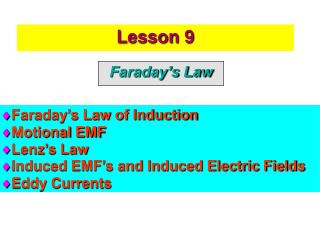Lesson 9

# Lesson 9 - PowerPoint PPT Presentation

Lesson 9. Lesson 9. Faraday’s Law. Faraday’s Law of Induction Motional EMF Lenz’s Law Induced EMF’s and Induced Electric Fields Eddy Currents. Torque on Loop. Current in loop in a magnetic field produces torque on a loop. Induced Current.I am the owner, or an agent authorized to act on behalf of the owner, of the copyrighted work described.
Download Presentation## Lesson 9

An Image/Link below is provided (as is) to download presentation

Download Policy: Content on the Website is provided to you AS IS for your information and personal use and may not be sold / licensed / shared on other websites without getting consent from its author.While downloading, if for some reason you are not able to download a presentation, the publisher may have deleted the file from their server.

- - - - - - - - - - - - - - - - - - - - - - - - - - E N D - - - - - - - - - - - - - - - - - - - - - - - - - -
Presentation Transcript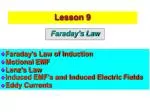Lesson 9

Lesson 9

• Faraday’s Law of Induction
• Motional EMF
• Lenz’s Law
• Induced EMF’s and Induced Electric Fields
• Eddy Currents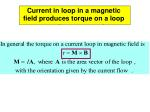Torque on Loop

Current in loop in a magnetic field produces torque on a loop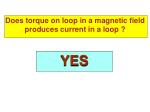Induced Current

Does torque on loop in a magnetic field produces current in a loop ?

YES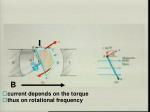Picture

I

B

• current depends on the torque
• thus on rotational frequency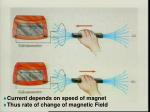Change of Flux Picture
• Current depends on speed of magnet
• Thus rate of change of magnetic Field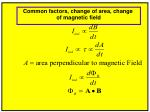Change of Flux Picture Equations

Common factors, change of area, change of magnetic field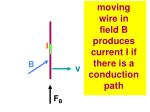Induced Current in Wire

moving wire in field B produces current I if there is a conduction path

I

B

v

FB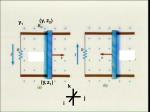Induced emf

(y,

z1)

(y,

z2)

y1

k

j

i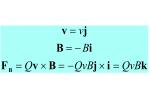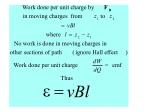Equations II

Work done per unit charge by

F

B

in moving charges from

z

to

z

1

2

=

vBl

where

l

=

z

-

z

2

1

No work is done in moving charges in

other sections of path

(

ignore Hall effect

)

dW

Work done per unit charge

=

emf

dQ

Thus

e

=

vBlEquations III

Area of loop in magnetic field

(

)

(

)

(

)

A

t

=

y

t

-

y

l

1

Total magnetic flux through loop

(

)

F

t

Rate of change of magnetic flux

e

d

F

dy

=

-B

l

=

-

Bvl

=

-

dt

dts Law of Induction for N loops'

Faradays Law of Electromagnetic Induction

The work done per unit charge by magnetic force

moving charge from

z

to

z

1

2

ò

ò

ò

z

2

dW

1

1

=

·

=

·

=

·

F

s

F

s

E

s

d

d

d

B

B

ind

dQ

Q

Q

z

loop

loop

1

thus

ò

e

F

d

=

-

=

·

d

E

s

N

ind

dt

loopInduced Electric Field
• An induced EMF is a measure of
• An induced Electric Field
• If charge is in this region and there is a conduction path it will feel a force from the induced Electric Field and flowEquations

E

Remember for a static electric field

stat

ò

b

=

·

E

s

V

d

and

ab

stat

a

ò

=

·

=

E

s

E

d

0

as

is conservative

stat

stat

E

But for an induced electric field

ind

ò

·

¹

E

s

d

0

ind

thus

E

is not conservative

indMagnetic Flux and Induced Electric Field

Changing Magnetic Flux produces an

Induced Electric FieldMechanical Work to Electrical work I

Pulling at constant velocity v

B

v

l

Fappl

I

Blv

y

k

j

iMechanical Work to Electrical work II

l

wire

with current I flowing in it

B

moving in a magnetic field

feels a force given by

=

´

F

l

B

I

=

-

´

=

-

F

k

i

j

IlB

IlB

F

This force opposes the applied force

appl

and must be equal and opposite if the

velocity is to remain constant

=

=

F

F

IlB

applMechanical Power to Electrical Power II

Pulling at constant velocity v

B

v

F

l

Fappl

I

BlvMagnetic Field produced by Changing Current

Circulating current produces an induced magnetic field

I

Bind

That opposes the external magnetic field B

That produces the currentCurrent produced by Changing Magnetic Field

(a) Change of External Magnetic Field Produces Current

(b) Current Produces Induced Magnetic FieldLenz's Law

Lenz’s Law

Polarity of is such that

it opposes the change that caused it

Direction of Eis such that

it opposes the change that caused it

Direction of induced current is such that

it opposes the change that caused itConservation of Energy

Conservation of EnergyAC Generator

AC Generatore

AC Potential

F

d

d

(

)

(

)

=

-

=

-

·

B

A

t

dt

dt

d

(

)

(

)

=

-

=

q

BACos

BA

sin

d

q

(

t

)

q

t

dt

dt

if rotational speed is constant

e

e

(

)

(

)

(

)

w

w

t

wBA

sin

t

sin

t

=

=

max

e

=

BAw

maxDC Generator

DC Generator